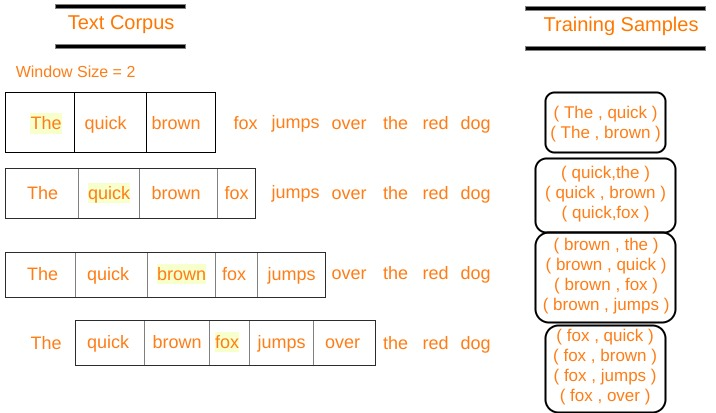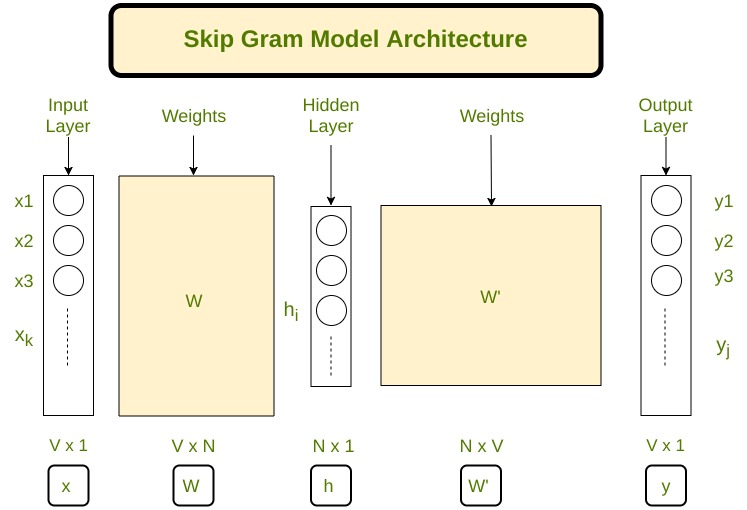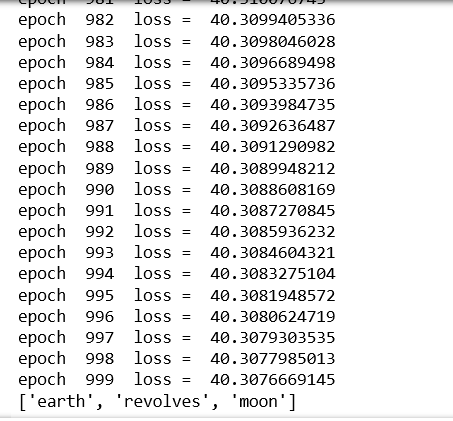GeeksforGeeks App
Open AppBrowser
Continue

# Implement your own word2vec(skip-gram) model in Python

Prerequisite: Introduction to word2vec
Natural language processing (NLP) is a subfield of computer science and artificial intelligence concerned with the interactions between computers and human (natural) languages.
In NLP techniques, we map the words and phrases (from vocabulary or corpus) to vectors of numbers to make the processing easier. These types of language modeling techniques are called word embeddings
In 2013, Google announced word2vec, a group of related models that are used to produce word embeddings.
Let’s implement our own skip-gram model (in Python) by deriving the backpropagation equations of our neural network.
In skip-gram architecture of word2vec, the input is the center word and the predictions are the context words. Consider an array of words W, if W(i) is the input (center word), then W(i-2), W(i-1), W(i+1), and W(i+2) are the context words if the sliding window size is 2.Let's define some variables :

V    Number of unique words in our corpus of text ( Vocabulary )
x    Input layer (One hot encoding of our input word ).
N    Number of neurons in the hidden layer of neural network
W    Weights between input layer and hidden layer
W'   Weights between hidden layer and output layer
y    A softmax output layer having probabilities of every word in our vocabularySkip gram architecture

Our neural network architecture is defined, now let’s do some math to derive the equations needed for gradient descent.

### Forward Propagation:

Multiplying one hot encoding of the center word (denoted by x) with the first weight matrix W to get hidden layer matrix h (of size N x 1).( Vx1 )        ( NxV )   ( Vx1 )
Now we multiply the hidden layer vector h with second weight matrix W’ to get a new matrix u( Vx1 )        ( VxN )   ( Nx1 )
Note that we have to apply a softmax> to layer u to get our output layer y.
Let uj be jth neuron of layer u
Let wj be the jth word in our vocabulary where j is any index
Let Vwj be the jth column of matrix W’(column corresponding to a word wj)( 1×1 )        ( 1xN )   ( Nx1 )
y = softmax(u)
yj = softmax(uj
yj denotes the probability that wj is a context wordP(wj|wi) is the probability that wj is a context word, given wi is the input word.
Thus, our goal is to maximize P( wj* | wi ), where j* represents the indices of context words
Clearly, we want to maximizewhere j*c are the vocabulary indexes of context words. Context words range from c = 1, 2, 3..C
Let’s take a negative log-likelihood of this function to get our loss function, which we want to minimizeLet t be the actual output vector from our training data, for a particular center word. It will have 1’s at the positions of context words and 0’s at all other places. tj*c are the 1’s of the context words.
We can multiplywithSolving this equation we get our loss function as –### Back Propagation:

The parameters to be adjusted are in the matrices W and W’, hence we have to find the partial derivatives of our loss function with respect to W and W’ to apply the gradient descent algorithm.
We have to findNow, FindingBelow is the implementation :

## Python3

 import numpy as npimport stringfrom nltk.corpus import stopwords  def softmax(x):    """Compute softmax values for each sets of scores in x."""    e_x = np.exp(x - np.max(x))    return e_x / e_x.sum()  class word2vec(object):    def __init__(self):        self.N = 10        self.X_train = []        self.y_train = []        self.window_size = 2        self.alpha = 0.001        self.words = []        self.word_index = {}      def initialize(self,V,data):        self.V = V        self.W = np.random.uniform(-0.8, 0.8, (self.V, self.N))        self.W1 = np.random.uniform(-0.8, 0.8, (self.N, self.V))                  self.words = data        for i in range(len(data)):            self.word_index[data[i]] = i            def feed_forward(self,X):        self.h = np.dot(self.W.T,X).reshape(self.N,1)        self.u = np.dot(self.W1.T,self.h)        #print(self.u)        self.y = softmax(self.u)         return self.y              def backpropagate(self,x,t):        e = self.y - np.asarray(t).reshape(self.V,1)        # e.shape is V x 1        dLdW1 = np.dot(self.h,e.T)        X = np.array(x).reshape(self.V,1)        dLdW = np.dot(X, np.dot(self.W1,e).T)        self.W1 = self.W1 - self.alpha*dLdW1        self.W = self.W - self.alpha*dLdW              def train(self,epochs):        for x in range(1,epochs):                   self.loss = 0            for j in range(len(self.X_train)):                self.feed_forward(self.X_train[j])                self.backpropagate(self.X_train[j],self.y_train[j])                C = 0                for m in range(self.V):                    if(self.y_train[j][m]):                        self.loss += -1*self.u[m]                        C += 1                self.loss += C*np.log(np.sum(np.exp(self.u)))            print("epoch ",x, " loss = ",self.loss)            self.alpha *= 1/( (1+self.alpha*x) )                 def predict(self,word,number_of_predictions):        if word in self.words:            index = self.word_index[word]            X = [0 for i in range(self.V)]            X[index] = 1            prediction = self.feed_forward(X)            output = {}            for i in range(self.V):                output[prediction[i]] = i                          top_context_words = []            for k in sorted(output,reverse=True):                top_context_words.append(self.words[output[k]])                if(len(top_context_words)>=number_of_predictions):                    break                  return top_context_words        else:            print("Word not found in dictionary")

## Python3

 def preprocessing(corpus):    stop_words = set(stopwords.words('english'))       training_data = []    sentences = corpus.split(".")    for i in range(len(sentences)):        sentences[i] = sentences[i].strip()        sentence = sentences[i].split()        x = [word.strip(string.punctuation) for word in sentence                                     if word not in stop_words]        x = [word.lower() for word in x]        training_data.append(x)    return training_data        def prepare_data_for_training(sentences,w2v):    data = {}    for sentence in sentences:        for word in sentence:            if word not in data:                data[word] = 1            else:                data[word] += 1    V = len(data)    data = sorted(list(data.keys()))    vocab = {}    for i in range(len(data)):        vocab[data[i]] = i          #for i in range(len(words)):    for sentence in sentences:        for i in range(len(sentence)):            center_word = [0 for x in range(V)]            center_word[vocab[sentence[i]]] = 1            context = [0 for x in range(V)]                         for j in range(i-w2v.window_size,i+w2v.window_size):                if i!=j and j>=0 and j<len(sentence):                    context[vocab[sentence[j]]] += 1            w2v.X_train.append(center_word)            w2v.y_train.append(context)    w2v.initialize(V,data)      return w2v.X_train,w2v.y_train

## Python3

 corpus = ""corpus += "The earth revolves around the sun. The moon revolves around the earth"epochs = 1000 training_data = preprocessing(corpus)w2v = word2vec() prepare_data_for_training(training_data,w2v)w2v.train(epochs) print(w2v.predict("around",3))

Output:My Personal Notes arrow_drop_up